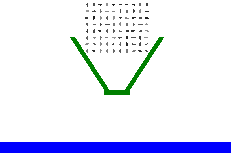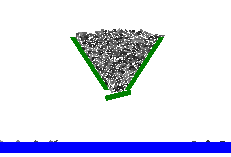SYSTEMS » UNIVERSAL MECHANISM » UM Granular Media

# UM Granular Media

Introduction to UM Ballast

This module allows including in a UM model a planar granular media, which is a set of particles (rigid polygons and circles). The particles interact with each other by contact forces. Simple models of contact forces are used in the module. Each force consists of two components. The first component is a linear viscoelastic normal force. Another one is a dry friction force with two modes: sliding and sticking. Also the particles interact with other bodies (walls, bottom of a box, sieves and so on). In term of UM any granular media is a subsystem. That is why the UM Subsystems module is necessary for working in UM Ballast. Statistics of geometrical characteristics of particles are used for granular system creating.

During simulation a set of output parameters can be obtained such as:

• porosity of a granular media (local and global);

• distribution of velocities of the particles in the volume;

• distribution of loading of the particles in the volume;

• reactions of the media on the walls and bottom and graphics of the whole set of variables for each particle (trajectories, velocities, accelerations and so on).Detraining from hopper car IDetraining from hopper car Ii

Overview of existing approaches to simulation of granular media

There are several principally different approaches to this problem.

The major ones are: simulation of granular media (GM) as a continuous system, for example,

using the finite element method;

simulation of the GM as a rigid model.

Overview of existing approaches to simulation of granular media

There are several principally different approaches to this problem. The major ones are:

• simulation of granular media (GM) as a continuous system, for example, using the finite element method;
• simulation of the GM as a rigid model.

The first approach is limited in applying due to the fact that it is not directly take into account the geometry of the particles of the ballast. But the second approach requires a much higher computational costs in the usual formulation. In the present module (UM Ballast) implements the second approach.

Decreasing the number of arithmetic operations mainly is associated with search and implementation of the fast algorithm for determination of colliding bodies in their position. Increasing the integration step is determined by the choice of the most successful method of numerical integration of stiff ordinary differential equations and the search for the representation of the Jacobian matrix of equations of motion required for implementation of implicit schemes for the numerical method.

The basis of the contact interaction is a model in which the contact interaction forces only occurs at the vertices of the limiting polygons at their penetration into the polygon that restricts neighboring granule. The force of contact interaction, rather, a normal component, depends on penetration depth and speed of implementation. The penetration is calculated as the minimum distance from the implemented top to the sides of the polygon. In the presence of sharp corners in the contours of polygons specified model can cause incorrect results, so the rough edges "cut off" in small neighborhoods of the vertices. Because the contact force depends of the distance between the top and one side of a polygon, it called the interaction "point-line" contact type. In fact, this interaction is a special case of spatial interaction "point-to-plane".

Contact force consists of two components: a normal reaction normal to the contact plane and friction forces, lying in this plane. As in the case of contact "point-to-plane", we assume a linear viscoelastic model of the normal force with constant coefficients of the contact stiffness and dissipation.

There are several different methods for determining contact of the polygons. Almost always, the problem is divided into two distinct parts: the definition of the contact membranes outlined around of the polygons ("remote" contact) and the refinement of contact, taking into account the real geometry ("close" contact). In turn, these two problems are also solved in several ways. For "long" contact, for example, you can choose the shell as a circle, and then the definition of the intersection is reduced to the trivial verification conditions. The main disadvantage of this method is the need to check this condition at each step for each pair of bodies, that is, the method is quadratic in the number of bodies. Method of coupled linear lists (Linked Linear List) is much faster and more linear in the number of bodies. This method used in present module.

There are several methods for determine the "close" contact, i.e. touching of polygons . One of the fastest of them are LinKenny algorithm and a method of beam. Lin Kenny algorithm based on the use of the “areas of Voronov”. This method can effectively determine the distance between the polygons. Unfortunately, the application of this algorithm is effective only in the case of convex polygons, and can not be used for determining the intersection of polygons. Therefore, it is ideal for modeling contact forces on the basis of the classical theory of impact of a small number of bodies. The second method determines the fact of the point hit into the polygon by the parity of the number of intersections of the ray passing through the top with the sides of the polygon. However, along with the definition of fact that the point hit into the polygon, definition of penetration is required. In the described module implements an alternative algorithm that combines both of these methods and is faster, it is the “method of sensitivity zones”.